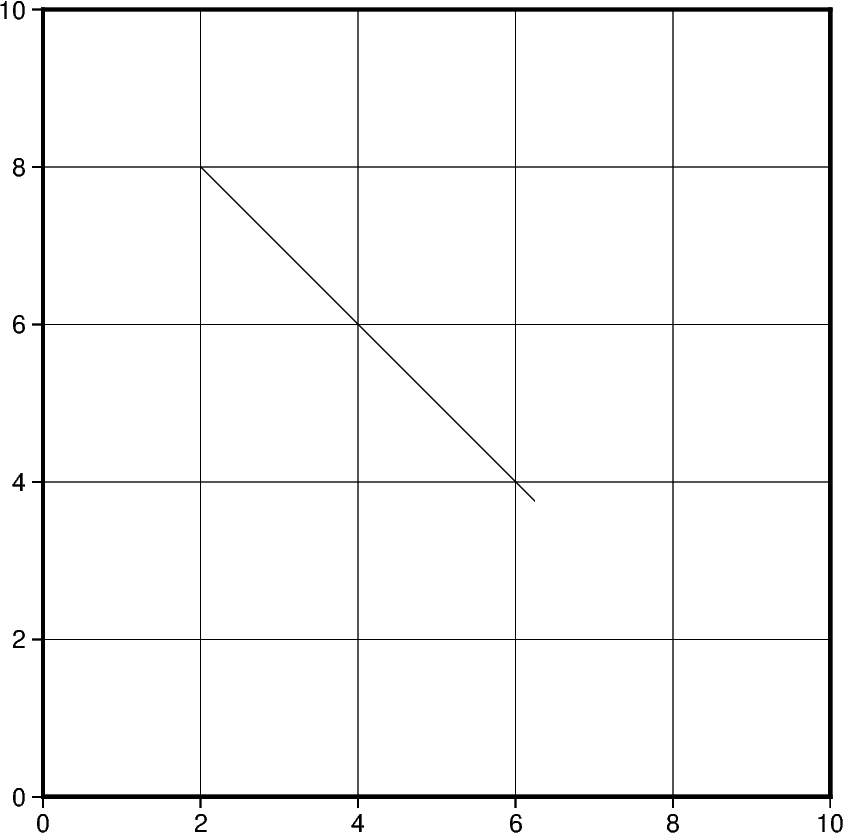# Cartesian, circular, and geographic vectors¶

The `pygmt.Figure.plot` method can plot Cartesian, circular, and geographic vectors. The `style` parameter controls vector attributes. See also Vector attributes documentation.Out:

```<IPython.core.display.Image object>
```

```import numpy as np
import pygmt

# create a plot with coast, Mercator projection (M) over the continental US
fig = pygmt.Figure()
fig.coast(
region=[-127, -64, 24, 53],
projection="M15c",
frame=True,
borders=1,
area_thresh=4000,
shorelines="0.25p,black",
)

# Left: plot 12 Cartesian vectors with different lengths
x = np.linspace(-116, -116, 12)  # x vector coordinates
y = np.linspace(33.5, 42.5, 12)  # y vector coordinates
direction = np.zeros(x.shape)  # direction of vectors
length = np.linspace(0.5, 2.4, 12)  # length of vectors
# Cartesian vectors (v) with red pen and fill (+g, +p), vector head at end (+e),
# and 40 degree angle (+a) with no indentation for vector head (+h)
style = "v0.2c+e+a40+gred+h0+p1p,red"
fig.plot(x=x, y=y, style=style, pen="1p,red", direction=[direction, length])
fig.text(text="CARTESIAN", x=-112, y=44.2, font="13p,Helvetica-Bold,red", fill="white")

# Middle: plot 7 math angle arcs with different radii
num = 7
x = np.full(num, -95)  # x coordinates of the center
y = np.full(num, 37)  # y coordinates of the center
radius = 1.8 - 0.2 * np.arange(0, num)  # radius
startdir = np.full(num, 90)  # start direction in degrees
stopdir = 180 + 40 * np.arange(0, num)  # stop direction in degrees
# data for circular vectors
data = np.column_stack([x, y, radius, startdir, stopdir])
arcstyle = "m0.5c+ea"  # Circular vector (m) with an arrow at end
fig.plot(data=data, style=arcstyle, color="red3", pen="1.5p,black")
fig.text(text="CIRCULAR", x=-95, y=44.2, font="13p,Helvetica-Bold,black", fill="white")

# Right: plot geographic vectors using endpoints
NYC = [-74.0060, 40.7128]
CHI = [-87.6298, 41.8781]
SEA = [-122.3321, 47.6062]
NO = [-90.0715, 29.9511]
# `=` means geographic vectors.
# With the modifier '+s', the input data should contain coordinates of start and end points
style = "=0.5c+s+e+a30+gblue+h0.5+p1p,blue"
data = np.array([NYC + CHI, NYC + SEA, NYC + NO])
fig.plot(data=data, style=style, pen="1.0p,blue")
fig.text(
text="GEOGRAPHIC", x=-74.5, y=44.2, font="13p,Helvetica-Bold,blue", fill="white"
)
fig.show()
```

Total running time of the script: ( 0 minutes 2.254 seconds)

Gallery generated by Sphinx-Gallery